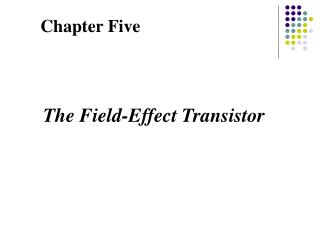Download PresentationChapter Five

Loading in 2 Seconds...

# Chapter Five - PowerPoint PPT Presentation

Chapter Five. The Field-Effect Transistor. Figure 6—2I am the owner, or an agent authorized to act on behalf of the owner, of the copyrighted work described.
Download Presentation## Chapter Five

An Image/Link below is provided (as is) to download presentation

Download Policy: Content on the Website is provided to you AS IS for your information and personal use and may not be sold / licensed / shared on other websites without getting consent from its author.While downloading, if for some reason you are not able to download a presentation, the publisher may have deleted the file from their server.

- - - - - - - - - - - - - - - - - - - - - - - - - - E N D - - - - - - - - - - - - - - - - - - - - - - - - - -
Presentation Transcript
1. Chapter Five The Field-Effect Transistor

2. Figure 6—2 A three-terminal nonlinear device that can be controlled by the voltage at the third terminal vG: (a) biasing circuit; (b) I–V characteristic and load line. If vG = 0.5 V, the d-c values of ID and VD are as shown by the dashed lines.

3. Figure 6—3 Simplified cross-sectional view of a junction FET: (a) transistor geometry; (b) detail of the channel and voltage variation along the channel with VG = 0 and small ID.

4. Figure 6—4 Depletion regions in the channel of a JFET with zero gate bias for several values of VD : (a) linear range; (b) near pinch-off; (c) beyond pinch-off.

5. Figure 6—5 Effects of a negative gate bias: (a) increase of depletion region widths with VG negative; (b) family of current–voltage curves for the channels as VG is varied.

6. Figure 6—11 n-channel MOSFET cross-sections under different operating conditions: (a) linear region for VG > VT and VD < (VG2VT); (b) onset of saturation at pinch-off, VG > VT and VD = (VG2VT); (c) strong saturation, VG > VT and VD > (VG 2 VT ).

7. Figure 6—27 Drain current–voltage characteristics for enhancement transistors: (a) for n-channel VD, VG, VT, and ID are positive; (b) for p-channel all these quantities are negative.

8. Figure 6—28 Linear region transfer characteristics: (a) plot of drain current versus gate voltage for MOSFETs in the linear region; (b) transconductance as a function of gate bias.

9. Figure 6—29 Saturation region transfer characteristics: plot of square root of the drain current versus gate voltage for MOSFETs.

10. Figure 6—39 Equivalent circuit of a MOSFET, showing the passive capacitive and resistive components. The gate capacitance Ci is the sum of the distributed capacitances from the gate to the source-end of the channel (CGS) and the drain-end (CGD). In addition, we have an overlap capacitance (where the gate electrode overlaps the source/drain junctions) from the gate-to-source (COS) and gate-to-drain (COD). COD is also known as the Miller overlap capacitance. We also have p-n junction depletion capacitances associated with the source (CJS) and drain (CJD). The parasitic resistances include the source/drain series resistances (RS and RD), and the resistances in the substrate between the bulk contact and the source and drain (RBS and RBD). The drain current can be modeled as a (gate) voltage-controlled constant-current source.

11. Figure 5.24 (a) An NMOS common-source circuit and (b) the NMOS circuit for Example 5.3

12. Figure 5.25 (a) A PMOS common-source circuit, (b) results when saturation-region bias assumption is incorrect, and (c) results when nonsaturation-region bias assumption is correct

13. Figure 5.28 Transistor characteristics, vDS (sat) curve, load line, and Q-point for the NMOS common-course circuit in Figure 5.24 (b)

14. Figure 5.29 NMOS common-source circuit with source resistor

15. Figure 5.35 Circuit with enhancement load devices and NMOS driver

16. Figure 5.36 Voltage transfer characteristics of NMOS inverter with enhancement load device

17. Figure 5.37 (a) Depletion-mode NMOS device with the gate connected to the source and (b) current-voltage characteristics

18. Figure 5.39 Circuit with depletion load device and NMOS driver

19. Figure 5.40 Voltage transfer characteristics of NMOS inverter with depletion load device

20. Figure 5.47 (a) An NMOS common-source circuit with a time-varying signal coupled to the gate and (b) transistor characteristics, load line, and superimposed sinusoidal signals

21. Chapter Six Basic FET Amplifiers

22. Figure 6.13 Common-source circuit with voltage divider biasing and coupling capacitor

23. Figure 6.14 Small-signal equivalent circuit, assuming coupling capacitor acts as a short circuit

24. Figure 6.17 DC load line and transition point for NMOS circuit shown in Figure 6.16

25. Figure 6.19 Small-signal equivalent circuit of NMOS common-source amplifier with source resistor

26. Figure 6.28 NMOS source-follower or common-drain amplifier

27. Figure 6.29 (a) Small-signal equivalent circuit of NMOS source-follower and (b) small-signal equivalent circuit of NMOS source-follower with all signal grounds at a common point

28. Figure 6.34 Common-gate circuit

29. Figure 6.35 Small-signal equivalent circuit of common-gate amplifier

30. Figure 6.39 (a) NMOS amplifier with enhancement load device; (b) driver transistor characteristics and enhancement load curve with transition point

31. Figure 6.39c Voltage transfer characteristics of NMOS amplifier with enhancement load device

32. Figure 6.43 (a) NMOS amplifier with depletion load device; (b) driver transistor characteristics and depletion load curve, with transition points

33. Figure 6.43c (c) voltage transfer characteristics

34. Figure 6.45 (a) CMOS common-source amplifier; (b) PMOS active load i-v characteristic, (c) driver transistor characteristics with load curve, (d) voltage transfer characteristics

35. Figure 6.50 NMOS cascode circuit

36. Figure 6.52 Small-signal equivalent circuit of NMOS cascode circuit